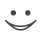# Get these flashcards, study & pass exams. For free! Even on iPhone/Android!

## Chem 2 (23 Cards)

Uncertainty
when measuring quantity every digit is certain except for the last.
style of writing numbers that consist of a decimal part and an exponential part
Scientific notation
Part of scientific notation that is a number usually between 1 and 10
decimal part
Part of scientific notaion expressed as 10 raised to an exponent
exponential part
non-place-holding digits in a reported measurement
significant figures (digits)

system of units used in the united states
english system

system of units used by most contries in the world
metric system
Set of standard units agreed upon by scientist throughout the world
International system
multiplyer used in the SI system that changes the value of a unit by a power of ten
prefix multipler
Fraction constructed from two quantities known to be the equivalent
Conversion Factor
Visual outline that shows the strategic route required to solve a problem
solution map
Quantity of matter
Mass
power to which a number is raised
exponent
Standard unit of mass in the SI (international system)
kilogram (kg)
Unit of volume
liter (L)
Standard unit of time in the SI system
secoonds (s)
non numerical part of a measured Value
units
Ratio of the mass of a substance to it's volume
Density
Measure of space
Volume
Standard unit of length in the SI system
Meter
Standard unit of time in the SI system
secoonds (s)
Units
measured quantities have units.

international system unit SI
length=meter
mass=kilogram
time=sec

Density
d=m/V
density equals mass divided by volumeFlashcard set info:
Author: jmarino
Main topic: Chemistry
Topic: General
Published: 19.05.2010

Card tags:
All cards (23)
no tagsReport abuse